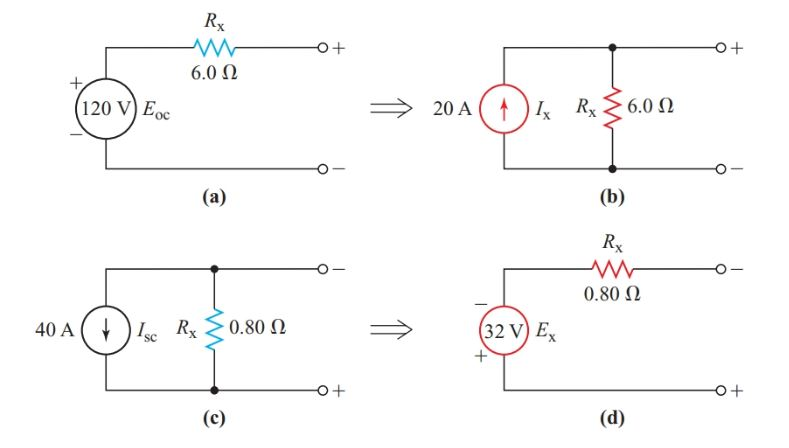# Source Transformation Example Problems with Solutions

Want create site? Find Free WordPress Themes and plugins.

Source transformation is a circuit analysis technique in which we convert voltage source in series with resistor into a current source in parallel with the resistor and vice versa.

For a given constant-voltage source, Rint in the equivalent constant-current source has the same value but appears in parallel with the ideal current source, as shown by the example in Figure 1.

Similarly, for a given constant-current source, Rint in the equivalent constant-voltage source has the same value but appears in series with the ideal voltage source.

In order for us to replace a constant-voltage source in a network with its constant-current equivalent, there must be some resistance Rx in series with an ideal voltage source.

Rx may be an internal resistance of the source, or it may be one of the network resistors in series with the voltage source.

Similarly, there must be some form of resistance in parallel with an ideal current source before we can replace it with its constant-voltage equivalent.

Applying Equation 1 to the constant-voltage source of Figure 1(a) shows that Ix for the equivalent constant-current source (Figure 1(b)) is the short-circuit current:

$\begin{matrix} {{\text{R}}_{\text{int}}}\text{=}\frac{\text{open circuit terminal voltage}}{\text{short circuit terminal current}} & {} & \left( \text{1} \right) \\\end{matrix}$

$\begin{matrix} {{I}_{X}}=\frac{{{E}_{oc}}}{{{R}_{X}}} & {} & \left( 2 \right) \\\end{matrix}$

Similarly, given the constant-current source of Figure 1(c), Ex for the equivalent constant-voltage source of Figure 1(d) is the open-circuit voltage:

$\begin{matrix} {{E}_{X}}={{I}_{sc}}{{R}_{X}} & {} & \left( 3 \right) \\\end{matrix}$Figure 1          Source transformation

## Source Transformation Example

(a)      Determine the equivalent constant-current source for a voltage source with an open-circuit voltage of 120 V and an internal resistance of 6.0 V.

(b) Determine the equivalent constant-voltage source for a current source with a constant current of 40 A and an internal resistance of 0.80 V.

(c)       Check that the equivalent sources in part (a) produce the same results when connected to a 12-V load.

Solution

• The internal resistance for the constant-current source is the same as for the voltage source: Rint 5 6.0 V

${{I}_{X}}=\frac{{{E}_{oc}}}{{{R}_{\operatorname{int}}}}=\frac{120V}{6\Omega }=20A$

• Rint for the constant-voltage equivalent source is the same as for the current source:

\begin{align} & {{R}_{\operatorname{int}}}=0.80\Omega \\ & {{E}_{X}}={{I}_{sc}}{{R}_{\operatorname{int}}}=40A\times 0.80\Omega =32V \\\end{align}

• When we connect a 12-V load to the voltage source of Figure 1(a),

${{I}_{L}}=\frac{{{E}_{X}}}{{{R}_{X}}+{{R}_{L}}}=\frac{120V}{6\Omega +12\Omega }=6.7V$

And

${{V}_{L}}=6.7A\times 12\Omega =80V$

When we connect a 12-V load to the current source of Figure 1(b),

\begin{align} & {{V}_{L}}={{I}_{X}}\times \frac{{{R}_{X}}\times {{R}_{L}}}{{{R}_{X}}+{{R}_{L}}}=20A\times \frac{6\times 12}{6+12}=80V \\ & {{I}_{L}}=\frac{{{V}_{L}}}{{{R}_{L}}}=\frac{80V}{12\Omega }=6.7A \\\end{align}

Once we are familiar with the concept, we can convert voltage sources to equivalent current sources (and vice versa) for network analysis purposes with just a bit of mental arithmetic. Analysis of transistor circuits often involves resistance networks containing both voltage and current sources. Such networks are usually easier to solve if we convert the sources so that they are either all voltage sources or all current sources.

Did you find apk for android? You can find new Free Android Games and apps.